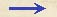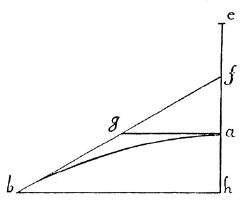Discorsi Propositions 3/05-pr-02 Discorsi Proposition3/05-pr-02PROPOSITIO V, PROBLEMA. PROPOSITION V, PROBLEMIn axe extenso datae parabolae punctum sublime reperire, ex quo cadens parabolam ipsam describit. Having given a parabola, find the point, in its axis extended upwards, from which a particle must fall in order to describe this same parabola. Sit parabola ab, cuius amplitudo hb, et axis extensus he, in quo reperienda sit sublimitas, ex qua cadens, et impetum in a conceptum in horizontalem convertens, parabolam ab describat.Ducatur horizontalis ag, quae erit parallela ipsi bh, et posita af {20} aequali ah, ducatur recta fb, quae parabolam tanget in b, et horizontalem ag in g secabit; accipiaturque ipsarum fa, ag tertia proportionalis ae: dico, e esse punctum sublime quaesitum, ex quo cadens ex quiete in e, et conceptum impetum in a in horizontalem convertens, superveniente impetu descensus in h ex quiete in a, parabolam ab describet.Si enim intelligamus, ea esse mensuram temporis descensus ex e in ab nec non impetus acquisiti in a, erit ag (media nempe inter ea, af) {30} tempus et impetus venientis ex f in a, seu ex a in h: et quia veniens ex e, tempore ea, cum impetu acquisito in a, conficit in latione horizontali, motus aequabili, duplam ea, ergo etiam latum eodem impetu conficiet in tempore ag duplam ga, mediam nempe bh (spatia enim confecta eodem motu aequabili sunt inter se ut eorundem motuum tempora), et in perpendiculari motu, ex quiete, eodem tempore ga, conficitur ah; ergo eodem tempore {294} conficiuntur a mobili amplitudo hb et altitudo ah.Describitur ergo parabola ab ex casu venientis a sublimate e: quod quaerebatur. Let ab be the given parabola, hb its amplitude, and he its axis extended. The problem is to find the point e from which a body must fall in order that, after the momentum which it acquires at a has been diverted into a horizontal direction, it will describe the parabola ab. Draw the horizontal ag, parallel to bh, and having laid off af equal to ah, draw the straight line bf which will be a tangent to the parabola at b, and will intersect the horizontal ag at g: choose e such that ag will be a mean proportional between af and ae. Now I say that e is the point above sought. That is, if a body falls from rest at this point e, and if the momentum acquired at the point a be diverted into a horizontal direction, and compounded with the momentum acquired at h in falling from rest at a, then the body will describe the parabola ab. For if we understand ea to be the measure of the time of fall from e to a, and also of the momentum acquired at a, (Condition 3/03-th-02) then ag (which is a mean proportional between ea and af) will represent the time and momentum of fall from f to a or, what is the same thing, from a to h; (Condition 23S1) and since a body falling from e, during the time ea, will, owing to the momentum acquired at a, traverse at uniform speed a horizontal distance which is twice ea, it follows that, the body will if impelled by the same momentum, during the time-interval ag traverse a distance equal to twice ag which is the half of bh. (Condition Impetus-veloc-prop) (Condition 1/01-th-01) This is true because, in the case of uniform motion, the spaces traversed vary directly as the times. And likewise if the motion be vertical and start from rest, the body will describe the distance ah in the {294} time ag. Hence the amplitude bh and the altitude ah are traversed by a body in the same time. (Condition 3/01-th-01) Therefore the parabola ab will be described by a body falling from the sublimity of e. Q. E. F.Discorsi Propositions 3/05-pr-02 Discorsi Proposition3/05-pr-02